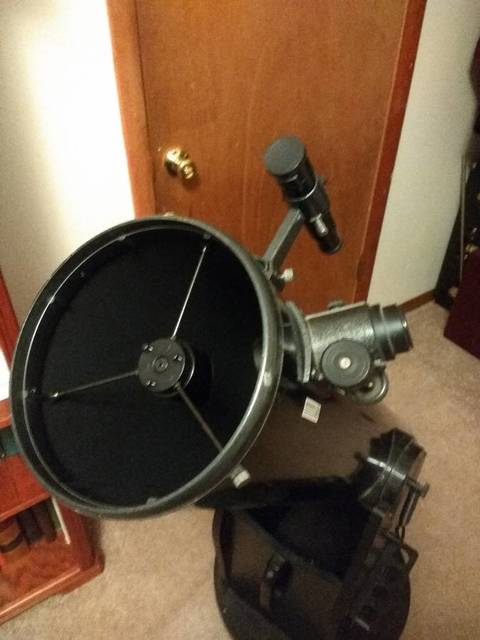# What the “f/ratio” Means in Telescope LingoTo understand what “f” means, it is imperative first to understand 2 main aspects of the telescope as it is mainly a relationship between these two.

• Aperture – it is the most important specification of any telescope. It is basically the measurement of the diameter of the main lens or mirror of the telescope.

The size of a telescope’s aperture determines its light collecting ability and is related by being directly proportional to an area of the lens or mirror, which is in turn associated with the square of the aperture.

For example, a telescope with an objective mirror of a 200mm aperture collects four times as much light as a scope with only a 100mm mirror.

• Focal Length – the focal length is the distance from the telescope’s optic lens to the plane in which the image comes into focus. The plane that is one focal length away from a lens or a mirror is called the focal plane.

This is where you can see the real image of a distant object. The focal length of the objective lens or mirror of a telescope influences the total length of the telescope.

The f/ratio in photography means the same for telescopes. It is the focal length of the telescope divided by its aperture. One of the most common telescope designs is a 120mm aperture (4.7”) refractor with a focal length of 1 meter (1000mm). In this case, the f/ratio is 1000/120 = 8.3  It is more conveniently written as “f/8.3”.

## A telescope’s f/ratio affects 3 things:

• Telescope Size – having a higher f/ratio means that your telescope has a longer focal length and telescope tube while a lower f/ratio means shorter focal length and telescope tubes.

This is because the focal length is proportional to the length of the telescope , or more specifically the distance between the lens and the focal point.

• Typical Magnification Range – a telescope of a given aperture and small f/ratio will give lower magnification with a given eyepiece than one with a larger f/ratio.
• Optical Distortion – telescopes with lower f/ratios can be affected greater by certain optical distortions or aberrations than those with higher f/ratios. They suffer from false color (“chromatic aberration”) around brighter objects and have significant distortions at the edges of the field of view.  This comes into play when the focal length is short compared to the diameter of the aperture.

## How Does the F Number Determine Your Magnification?

The telescope’s magnification is equal to the telescope’s focal length divided by the eyepiece’s focal length. For example, a telescope with a 1,200mm focal length that is used with a 20mm eyepiece, the magnification will be 1200/20, or 60x.

Unlike aperture, a higher magnification is not always better. There is a theoretical limit for the useful magnification of a given telescope, which is two times the aperture in millimeters. For a 120mm aperture, the theoretical limit is 240x. Beyond this limit, you will get a closer view, but the image will be fuzzy and dim.

## Is There a Focal ratio Better for Deep Space Viewing?

Different f/ratios are beneficial for different viewing purposes, so there is no one best f/ratio among all others. For example, a long focal ratio gives a higher magnification, but it also provides a narrower field of view. This is best for observing the moon, the planets, and the stars.

For these objects, the best f/ratio is f/10 or more. On the other hand, a lower focal ratio is best to see full views of star clusters, galaxies, and the Milky Way. They have less magnification, but they give you a broader view of the night sky.  Like the Dobsonian below.## What about Astrophotography, What f/ratio is Best Here?

For visual astronomy, the most basic rule is that the larger the aperture, the better. It makes sense because the aperture determines the light-gathering capability of your telescope and the higher this is, the finer details it can resolve. For astrophotography, however, what’s more important is the f/ratio rather than the aperture.

In cameras, the f/ratio is what determines how much light falls onto each of its pixels. We would want to maximize this because the objects we are imaging are usually very comparatively dim. The general rule is that the lower the f/ratio, the more light hits each pixel.

One could say it this way; the lower the f/ratio means that we gather light more quickly than that of a higher f/ratio.

## Need More?

As a note, the Hubble has an f ratio of F/24.  To learn more about the Hubble Space Telescope specs here on Wikipedia.

Plus, Here is my article on How To Rent Time on The Hubble Space Telescope

You may find more of the articles here note worthy too.  Let me know what you think…

What is a Refractor Telescope

30 Quick Tips & Tricks for Better Telescope Viewing

How Much Do Telescopes Weigh, a comparative guide